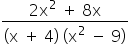Mathematics
Easy

Question

# For what value of x is the expression undefined?## -8-304Hint:

## The correct answer is: -3

### In mathematics, any number that can be written as p/q where q 0 is considered a rational number. Additionally, every fraction that has an integer denominator and numerator and a denominator that is not zero falls into the category of rational numbers. The outcome of dividing a rational number, or fraction, will be a decimal number, either a terminating decimal or a repeating decimal.Now we have given the expression as:The expression is undefined when denominator is zero, so:So at the values -4, -3 and 3, x is undefined.

So here the concept of rational numbers is used. When the denominator is 0, a rational expression has no specified meaning. Set the denominator to 0 and solve for x to get the values of x that lead to a denominator of 0. So at the values -4, -3 and 3, x is undefined.

### Related Questions to study#### With Turito Foundation.#### Get an Expert Advice From Turito.